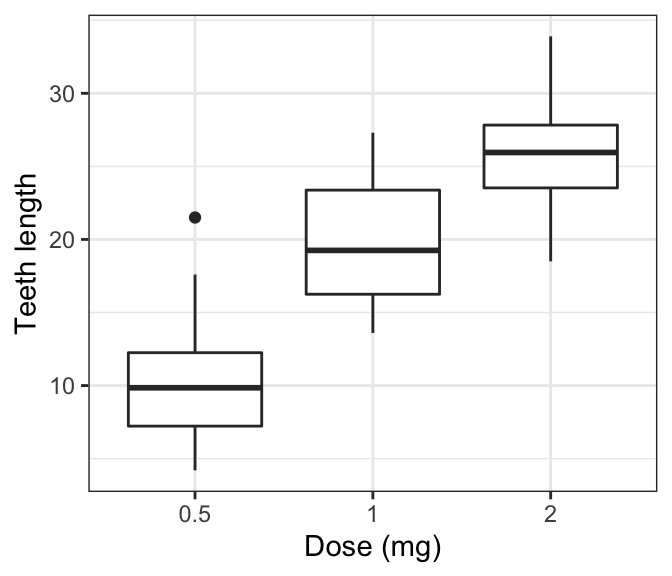# GGPlot Axis Labels#### GGPlot Axis Labels

This article describes how to change ggplot axis labels (or axis title). This can be done easily using the R function labs() or the functions xlab() and ylab().

In this R graphics tutorial, you will learn how to:

• Remove the x and y axis labels to create a graph with no axis labels. For example to hide x axis labels, use this R code: p + theme(axis.title.x = element_blank()).
• Change the font style of axis labels (size, color and face).

Contents:

## Key ggplot2 R functions

• Start by creating a box plot using the ToothGrowth data set:
library(ggplot2)
p <- ggplot(ToothGrowth, aes(x = factor(dose), y = len)) +
geom_boxplot()
• Change x and y axis labels as follow:
• p + xlab(“New X axis label”): Change the X axis label
• p + ylab(“New Y axis label”): Change the Y axis label
• p + labs(x = “New X axis label”, y = “New Y axis label”): Change both x and y axis labels
• Key ggplot2 theme options to change the font style of axis titles:
theme(
axis.title = element_text(),         # Change both x and y axis titles

axis.title.x = element_text(),       # Change x axis title only
axis.title.x.top = element_text(),   # For x axis label on top axis

axis.title.y = element_text(),       # Change y axis title only
axis.title.y.right = element_text(), # For y axis label on right axis
)

Arguments of the function element_text() includes:

• color, size, face, family: to change the text font color, size, face (“plain”, “italic”, “bold”, “bold.italic”) and family.
• lineheight: change space between two lines of text elements. Number between 0 and 1. Useful for multi-line axis titles.
• hjust and vjust: number in [0, 1], for horizontal and vertical adjustment of axis titles, respectively.
• hjust = 0.5: Center axis titles.
• hjust = 1: Place axis titles on the right
• hjust = 0: Place axis titles on the left
• To remove a particular axis title, use element_blank() instead of element_text(), for the corresponding theme argument.

For example to remove all axis titles, use this: p + theme(axis.title = element_blank()).

## Change axis labels

# Default plot
print(p)

# Change axis labels
p <- p + labs(x = "Dose (mg)", y = "Teeth length")
p## Change label size, color and face

• Key functions: theme() and element_text()
• Allowed values for axis titles font face: “plain”, “italic”, “bold” and “bold.italic”
p + theme(
axis.title.x = element_text(color = "blue", size = 14, face = "bold"),
axis.title.y = element_text(color = "#993333", size = 14, face = "bold")
)## Remove axis labels

Key function: use element_blank() to suppress axis labels.

p + theme(axis.title.x = element_blank(),
axis.title.y = element_blank())Remove all axis titles at once:

p + theme(axis.title = element_blank())

## Conclusion

Change a ggplot x and y axis titles as follow:

p + labs(x = " x labels", y = "y labels")+
theme(
axis.title.x = element_text(size = 14, face = "bold"),
axis.title.y = element_text(size = 14, face = "bold.italic")
)